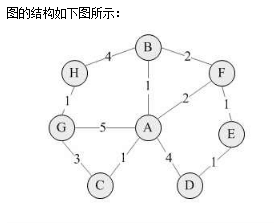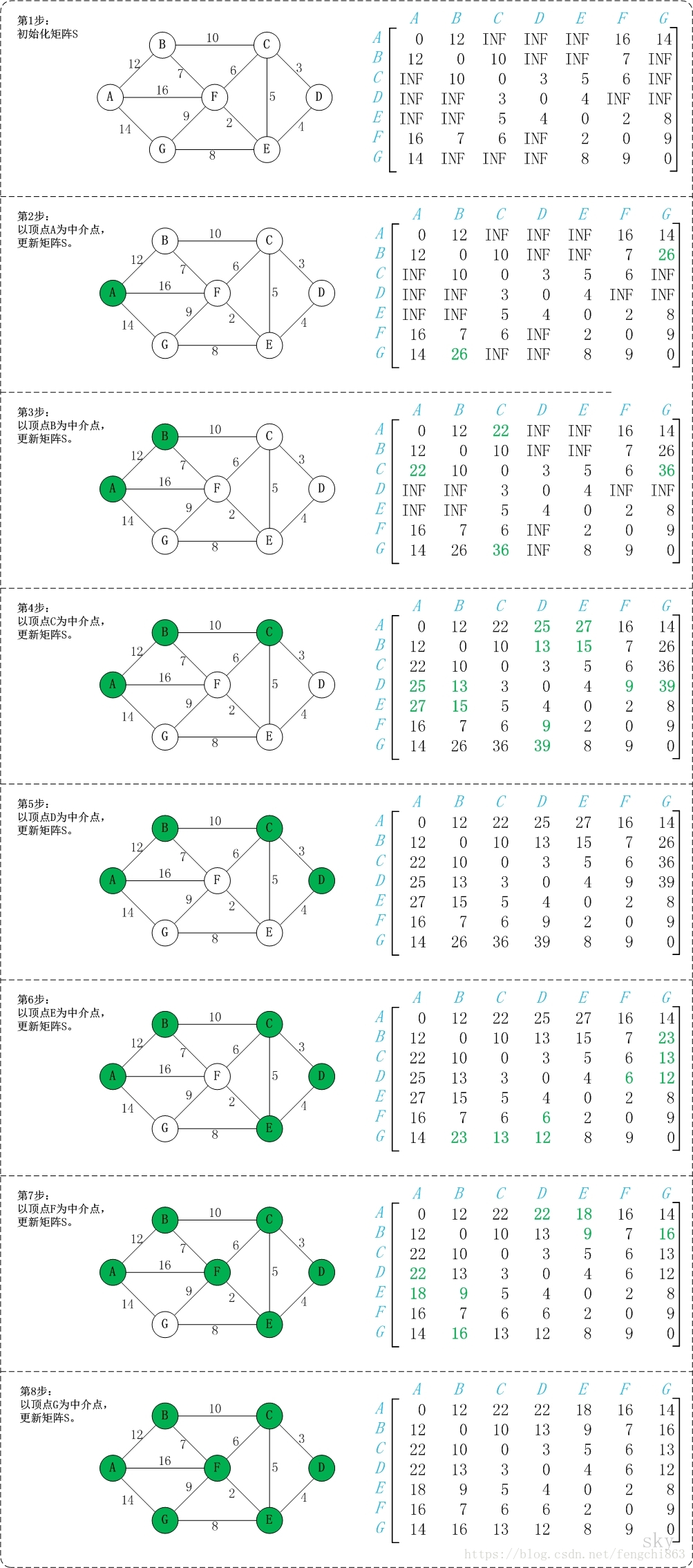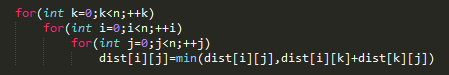• *有向网中的任意两点最短路径 *弗洛伊德算法的核心是 运用一个path二维数组 和一个A二维数组 *path数组用来保存中间路径经过的节点 *A数组用来保存任意两个顶点之间最短路径长度 *最后输出A数组运用了递归输出...
/* *求有向网中的任意两点的最短路径 *弗洛伊德算法的核心是 运用一个path二维数组 和一个A二维数组 *path数组用来保存中间路径经过的节点 *A数组用来保存任意两个顶点之间的最短路径长度 *最后输出A数组运用了递归输出的思想 *先初始化path数组和A数组 *运用三层循环 （算法的核心） 计算任意两点之间的最短路径长度 将经过的节点的下标存储在path数组中去 *递增的思想 *创建一个有向网 输出一个临接矩阵 */
#include<stdio.h>
#include<stdlib.h>
#include<string.h>
#define  OK 1
#define ERROR 0
#define   VERTEXNUM 100//最大顶点数
#define  NAME_SIZE 255//字符串的最大长度
#define MAX_INT 32726 //权重最大值
typedef int Statu;//返回值的数据类型
typedef  char*VertexType;//字符串的数据类型
typedef int ArcType;//权值数组的数据类型
typedef struct  amgraph
{
VertexType vexs[VERTEXNUM];//图的顶点数组
ArcType arcs[VERTEXNUM][VERTEXNUM];//图的权值的二维数组
int n;//图的顶点数
int e;//图的边数
}AMGraph;//图的结构体
int  path[VERTEXNUM][VERTEXNUM];//用于保存进过的中间路径的节点的下标
int A[VERTEXNUM][VERTEXNUM];//经过的路径节点的权值
void main()
{
test();//测试函数

}
void test();//测试函数
Statu create_DN(AMGraph*G);//创建有向网
int Locate_vex(AMGraph*G,VertexType vex);//定位函数
void Floyed_function(AMGraph*G);//弗洛伊德算法
void Dispath(int A[][VERTEXNUM],int path[][VERTEXNUM],int n);
//n代表当前图的顶点数
//最短路径第一输出函数  主要用于输出权值
void Ppath(int path[][VERTEXNUM],int i,int j);//用于输出路径节点的下标
//创建一个有向网
Statu create_DN(AMGraph*G)//创建有向网
{
int i,j;//循环变量
VertexType  vex1,vex2;//字符串的临时变量
int x,y;//定位函数的临时变量
int now_weight;//当前的权重
printf("请输入有向网的顶点数：");
scanf("%d",&G->n);
printf("请输入有向网的边数：");
scanf("%d",&G->e);
//初始化顶点的信息
for(i=0;i<G->n;i++)
{
G->vexs[i]=(VertexType)malloc(sizeof(char)*10);//动态申请存储空间
printf("顶点%d：",i+1);
scanf("%s",G->vexs[i]);

}
//初始化权值数组
for(i=0;i<VERTEXNUM;i++)
for(j=0;j<VERTEXNUM;j++)
{
G->arcs[i][j]=MAX_INT;//权值初始化

}
//初始化边的信息
for(i=0;i<G->e;i++)
{
vex1=(VertexType)malloc(sizeof(char)*10);//动态申请存储空间
vex2=(VertexType)malloc(sizeof(char)*10);//动态申请存储空间
printf("顶点：");
scanf("%s",vex1);
printf("邻接点：");
scanf("%s",vex2);
printf("请输入当前的权重：");
scanf("%d",&now_weight);
x=Locate_vex(G,vex1);
y=Locate_vex(G,vex2);
if(x==-1||y==-1)
{
return ERROR;
}
else
{
G->arcs[x][y]=now_weight;//创建的是有向网
}

}
return OK;
}
int Locate_vex(AMGraph*G,VertexType vex)
{
int index=0;
while(index<G->n)
{
if(strcmp(vex,G->vexs[index])==0)
{
break;
}
else
{
index++;
}
}
return (index==G->n ? -1 : index);
}
void Floyed_function(AMGraph*G)//弗洛伊德算法
{
int i,j;//循环变量
int k;
for(i=0;i<G->n;i++)
for(j=0;j<G->n;j++)
{
path[i][j]=-1;//path数组初始化
A[i][j]=G->arcs[i][j];
//初始化A数组  有边的存在 存储权值 没有边的存在 即为无穷大
}
//算法的核心  三层循环
for(k=0;k<G->n;k++)
for(i=0;i<G->n;i++)
for(j=0;j<G->n;j++)
if(A[i][j]>A[i][k]+A[k][j])
{
A[i][j]=A[i][k]+A[k][j];
path[i][j]=k;//存储路径

}

Dispath(A,path,G->n);//输出任意两个邻接点的最短路径长度

}
void Ppath(int path[][VERTEXNUM],int i,int j)//输出最短路径经过的中间节点
{
int k;
k=path[i][j];
if(k==-1)//两个顶点之间无中间节点
{
printf("%d->",i);
printf("%d",j);
}
else{
Ppath(path,i,k);//递归的思想   输出最短路径经过的节点
printf("%d->",k);
Ppath(path,k,j);}

}
void Dispath(int A[][VERTEXNUM],int path[][VERTEXNUM],int n)//依次输出最短路径的权值
{
int i,j;//循环变量
for(i=0;i<n;i++)
for(j=0;j<n;j++)
{
if(A[i][j]==MAX_INT)

if(i!=j)//排除顶点本身的情况
{
printf("从%d到%d没有路径\n",i,j);

}
else
{
printf("%d到%d的最短路径长度为\n",i,j);
// printf("path：");
printf("%d->",i);
Ppath(path,i,j);
printf("%d",j);
printf("路径长度为：%d\n",A[i][j]);
}

}

}

void test()
{
int i,j;//循环变量
AMGraph*G;
G=(AMGraph*)malloc(sizeof(AMGraph));
int result=create_DN(G);
if(result==ERROR)
{
printf("有向网创建失败！\n");
return ;
}
else//输出邻接矩阵
{
printf("该有向网的邻接矩阵为：\n");
printf("\t");
//输出第一行的顶点
for(i=0;i<G->n;i++)
{
printf("\t%s",G->vexs[i]);
}
printf("\n");//换行输出
//输出边的信息
for(i=0;i<G->n;i++)
{
printf("\t%s",G->vexs[i]);
for(j=0;j<G->n;j++)
{
if(G->arcs[i][j]==MAX_INT)
printf("\t∞");
else
printf("\t%d",G->arcs[i][j]);

}
printf("\n");//换行

}
}
printf("\n");
printf("最短路径及其经过的节点为:\n");
Floyed_function(G);

}

展开全文C语言数据结构
• // 保存start到其他各点最短路径的字符串表示 for (int i = 0; i ; i++){ path[i] = new String(point[start] + pathTip + point[i]); } int[] visited = new int[n]; // 标记当前该顶点的最短路径...
http://128kj.iteye.com/blog/1678532
package com.geo.xiaojinku.udf.utils;

import java.util.Map;
import java.util.Map.Entry;

import javax.print.attribute.standard.PrinterInfo;

public class FloydUtil {
private static int M = 10000; // 此路不通
private static String pathTip = "-->";
private static Map<String, Object> pathInfoMap = new LinkedHashMap<String, Object>(); // 路径和距离信息

public static void main(String[] args) {
int[][] weight1 = {
{ 0, 1, 1, 4, M, 2, 5, M },
{ 1, 0, M, M, M, 2, M, 4 },
{ 1, M, 0, M, M, M, 3, M },
{ 4, M, M, 0, 1, M, M, M },
{ M, M, M, 1, 0, 1, M, M },
{ 2, 2, M, M, 1, 0, M, M },
{ 5, M, 3, M, M, M, 0, 1 },
{ M, 4, M, M, M, M, 1, 0 }
};
String[] point = { "A", "B", "C", "D", "E", "F", "G", "H" };
for (int start = 0; start < point.length; start++) {
getPathInfo(weight1, start, point);
}
printPathInfo();

}
public static void getPathInfo(int[][] weight, int start, String[] point) {
// 接受一个有向图的权重矩阵，和一个起点编号start（从0编号，顶点存在数组中）
int n = weight.length; // 顶点个数
int[] shortPath = new int[n]; // 保存start到其他各点的最短路径
String[] path = new String[n]; // 保存start到其他各点最短路径的字符串表示
for (int i = 0; i < n; i++){
path[i] = new String(point[start] + pathTip + point[i]);
}
int[] visited = new int[n]; // 标记当前该顶点的最短路径是否已经求出,1表示已求出
// 初始化，第一个顶点已经求出
shortPath[start] = 0;
visited[start] = 1;
for (int count = 1; count < n; count++) { // 要加入n-1个顶点
int k = -1; // 选出一个距离初始顶点start最近的未标记顶点
int dmin = Integer.MAX_VALUE;
for (int i = 0; i < n; i++) {
if (visited[i] == 0 && weight[start][i] < dmin) {
dmin = weight[start][i];
k = i;
}
}
// 将新选出的顶点标记为已求出最短路径，且到start的最短路径就是dmin
shortPath[k] = dmin;
visited[k] = 1;
// 以k为中间点，修正从start到未访问各点的距离
for (int i = 0; i < n; i++) {
if (visited[i] == 0 && weight[start][k] + weight[k][i] < weight[start][i]) {
weight[start][i] = weight[start][k] + weight[k][i];
path[i] = path[k] + pathTip + point[i];
}
}
}
for (int i = 0; i < n; i++) {
// System.out.print("从" + point[start] + "出发到" + point[i] +
// "的最短路径为：" + path[i] + " ");
// System.out.println("从" + point[start] + "出发到" + point[i] +
// "的最短距离为：" + shortPath[i]);
Object[] objects = new Object;
objects = path[i];
objects = shortPath[i];
pathInfoMap.put(point[start] + pathTip + point[i], objects);
}

}
/**
* 打印路径信息和距离
*/
private static void printPathInfo() {
for (Entry<String, Object> entry : pathInfoMap.entrySet()) {
String key = entry.getKey();
Object[] objects = (Object[]) entry.getValue();
System.out.println(key + ":" + objects + "  路径长度：" + objects);
}
}

}展开全文• postgreSQL数据库用pgRouting求两点最短路径，详细描述操作步骤，直接复制可用
• 用C++图的最小生成树、单元点最短路径两点之间最短路径
• 给定一个乡镇网络图，个乡镇的最短路径，并输出路径，以及路径距离的大小。
• 一点有关任意两点最短路径的思考！！仅仅是个人拙见。
• Dijkstra算法是源点到其它顶点的最短路径。怎样任意个顶点之间最短路径
• 探路者 使用A *寻路找到两点之间最短路径的程序Python
• 所有两点之间的距离是边的权，如果两点之间没有边相连，则权为无穷大，这也是所谓的初始化工作； 对于每一对顶点 u 和 v，看看是否存在一个顶点 w 使得从 u 到 w 再到 v 比己知的路径更短。如果是更新它。 以下图为...
算法描述：
从任意一条单边路径开始。所有两点之间的距离是边的权，如果两点之间没有边相连，则权为无穷大，这也是所谓的初始化工作；对于每一对顶点 u 和 v，看看是否存在一个顶点 w 使得从 u 到 w 再到 v 比己知的路径更短。如果是更新它。
以下图为例，对Floyd 算法进行演示。图片转自https://blog.csdn.net/fengchi863/article/details/80036586
以下为c++版代码：
//

#include "stdafx.h"
#include<iostream>
#include<string>
#include<vector>
using namespace std;
const int N = 10;
const int INF = INT_MAX;
vector<int>v;
int mEdgNum;        // 边的数量
string mVexs[N];       // 顶点集合
int mMatrix[N][N];    // 邻接矩阵
int amount_path[N][N];

void floyd(string path[N][N], int dist[N][N], int length) {

// 初始化
for (int i = 0; i < length; i++) {
for (int j = 0; j < length; j++) {
dist[i][j] = mMatrix[i][j];    // "顶点i"到"顶点j"的路径长度为"i到j的权值"。
if (dist[i][j] != INF)
{
path[i][j] = mVexs[i] + mVexs[j];
amount_path[i][j] = 1;
}
else
path[i][j] = "";            // "顶点i"到"顶点j"的最短路径是经过顶点j。
}
}

// 计算最短路径
for (int k = 0; k < length; k++) {
for (int i = 0; i < length; i++) {
for (int j = 0; j < length; j++) {
// 如果经过下标为k顶点路径比原两点间路径更短，则更新dist[i][j]和path[i][j]
int tmp = (dist[i][k] == INF || dist[k][j] == INF) ? INF : (dist[i][k] + dist[k][j]);
int f = dist[i][j];
if (tmp < dist[i][j])
{
// "i到j最短路径"对应的值设，为更小的一个(即经过k)
dist[i][j] = tmp;
// "i到j最短路径"对应的路径，经过k
path[i][j] = path[i][k] + path[k][j];
amount_path[i][j] = 1;
}
else if (tmp == f&&k!=i&&k!=j)amount_path[i][j]++;
}
}
}
for (int i = 0; i < length; i++) {
for (int j = 0; j < length; j++)
if (i != j)
{
cout << "顶点" << i << "到顶点" << j << "的最短路径长度为" << dist[i][j] << endl;
cout << "路径为";
for (int k = 0; k < path[i][j].size() - 1; k++)
{
if (k == 0 && path[i][j][k] != path[i][j][k + 1])
cout << path[i][j][k]<<path[i][j][k+1];
else if (path[i][j][k] != path[i][j][k + 1])
cout << path[i][j][k+1];
}
cout <<endl;
cout << "最短路径条数为" << amount_path[i][j]<<endl;
}
}
}
int main()
{
int amount_vex, M;//M代表存在的路径数;
scanf_s("%d %d", &amount_vex, &M);
for (int i = 0; i < amount_vex; i++)//把每个点按数字由小到大编号
{
mVexs[i] = 48 + i;
}
string path[N][N];
int dist[N][N];
fill(amount_path,amount_path+amount_vex*amount_vex, 0);
for (int i = 0; i < amount_vex; i++)//初始化
{
for (int j = 0; j < amount_vex; j++)
{
if (i == j)mMatrix[i][j] = 0;
else mMatrix[i][j] = INF;
path[i][j] = "";
}
}
for (int i = 0; i < M; i++)
{
int m1, m2;
scanf_s("%d %d", &m1, &m2);
scanf_s("%d", &mMatrix[m1][m2]);
mMatrix[m2][m1] = mMatrix[m1][m2];
}

floyd(path, dist, amount_vex);

}


运行结果： 顶点0到顶点1的最短路径长度为1 路径为01 最短路径条数为1 顶点0到顶点2的最短路径长度为2 路径为02 最短路径条数为1 顶点0到顶点3的最短路径长度为3 路径为03 最短路径条数为2 顶点1到顶点0的最短路径长度为1 路径为10 最短路径条数为1 顶点1到顶点2的最短路径长度为3 路径为102 最短路径条数为1 顶点1到顶点3的最短路径长度为2 路径为13 最短路径条数为1 顶点2到顶点0的最短路径长度为2 路径为20 最短路径条数为1 顶点2到顶点1的最短路径长度为3 路径为201 最短路径条数为1 顶点2到顶点3的最短路径长度为2 路径为23 最短路径条数为1 顶点3到顶点0的最短路径长度为3 路径为30 最短路径条数为2 顶点3到顶点1的最短路径长度为2 路径为31 最短路径条数为1 顶点3到顶点2的最短路径长度为2 路径为32 最短路径条数为1 请按任意键继续. . .
dp版本：展开全文• 1 先出原点离中间之间最短路径，然后，基于已经出的最短路径，进一步出它们之间最短路径。 转载于:https://www.cnblogs.com/wwwfj/p/3350166.html

1 先求出原点离中间之间的最短路径，然后，基于已经求出的最短路径，进一步求出它们之间的最短路径。

转载于:https://www.cnblogs.com/wwwfj/p/3350166.html
展开全文• 通过输入，能够实现找到最短路径。源代码能运行，简单易懂
• 两点之间最短路径的计算-floyd算法 Floyd算法的核心内容 算法过程图解 伪代码 完整代码 void floyd() { for (int k = 0; k < n; ++k) { for (int i = 0; i < n; ++i) { for (int j = 0; j < n; +...
• 从P经过A、B、C、D、E、F、G、H、I后到达F，不必考虑这9个的相对顺序，P到J的最短路径
• MAX——定义两点之间若无路径赋予的最大值 变量： DIST[N]——存储已经搜寻到的最短路径 Is[N]——存储节点是否被遍历的状态 Path[N]——图之间的路径矩阵 Road[N]——存储最短路径时该节点的上一节点 算法...Dijkstra算法
• 用C语言编的求最短路径，用弗罗伊德算法，任意两点最短路径
• 如何才能两点之间最短路径呢？大家都知道学几何的时候，有一条定理就是：两点之间线段最短。但是在实际情况中，我往往两点之间没有之间的通路而是一些曲折的线路。 上面已经给出了两点之间的线路路径，不能再...
• 我使用spark的graphx图计算框架，现在要求一个图中所有节点对的最短路径条数， graphx下的pregel迭代貌似使用的是类迪杰斯特拉算法，要求最短路径长度很容易， 但是要求条数，我实在是想不出来怎么，希望各位大神...pregel spark
• 本文主要讲述最短路径算法，一个主要原因是网上的“基于Matlab实现的两点之间最短路径算法”存在各种实现错误，目前为止还没有找到一个完全正确的。所以，本人改正相关错误，上传个正确的版本，即：采用Matlab实现的...
• 1.求两点之间最短路径： （1）从某个源点到其余各点的最短路径：Dijstra（迪杰斯特拉）算法； （2）每一对顶点之间最短路径：Floyd（弗洛伊德）算法。 2.Dijstra算法的基本思想：依据最短路径的长度递增...
• 最短路径问题是经典图论问题之一。从工程意义上讲，最短路径问题是对大量工程问题的直观抽象。 最典型的例子是在地图上寻找最短驾车路径。 寻找从A到D的最短路径。 测试用例 用例1： 输入： 5,7 A,B,C,E,D ...
• 基于pgrouting任意两点最短路径的函数pgr_fromAtoBpgrouting
• 我们之前做的dijkstra算法只能实现两点之间的1条最短路径的计算。dijkstra算法需要和yen算法结合，才能实现获取两点之间的k条最短路径。关于后面的数据准备有疑惑的，可以参考上篇博文WebGIS开发之最短路径分析入门 ...dijkstra postgis
• 7*5矩阵方格中，红色A绕过障碍物到达B，移动规则： 1.A向周围8个小方格移动但是不能移动到旁边有球的方格 2.A球需要用最短路径到达B ：用java实现该算法
• Input先输入一个小于100的正整数n，然后输入图的邻接矩阵（10000表示无穷大，即两点之间没有边），最后输入两个0到n-1的整数表示两个点。Output先用迪杰斯特拉算法给定的第一个点到其余所有结点的最短路径。然后再...dijstra
• 最短路径算法1.Dijkstra算法2.Bellman-Ford算法3.SPFA算法4.Floyd算法几种最短路径算法的对比Dijkstra算法、Bellman-Ford算法和SPFA算法的对比Dijkstra算法和Floyd算法的...多源最短路算法：任意两点之间最短路径.算法
• 从文件读取图，然后寻找指定两点最短路径，并把寻找结果存入文件中，路径包括一条主路径和备用路径
• 图中任意两点最短路径及其大小 function [P u]=n2shorf(W,k1,k2) W是邻接矩阵，k1 k2分别是任意两点 P是最短路径 u是最短路径大小
• （Java）求两顶点间最短路径和距离 在网上查看了一些博客，发现他们的代码都有些问题，于是自己重新写了一个，有一定注释
• 要利用rgrouting实现像QGIS那样任意两点间的最短路径，可以按照以下步骤使用pl/pgsql进行自定义函数： 1 函数的参数为：_myShortPath(startxfloat, starty float,endx float,endy float,costfile varchar)，前四个...postgresql pgrouting postgis
• 注意：这里需要求解的最短路径指的是个城市之间的最短距离，而不是所有城市之间最短总距离。 1.最短路径算法 //最短路径算法 static void distMin(GraphMatrix GM,int vend){ //vend为结束......